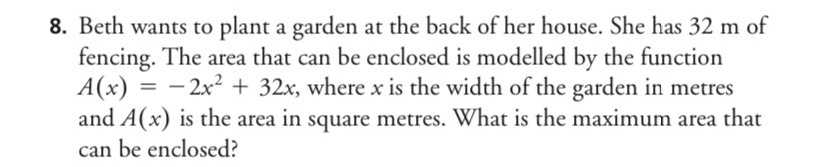### ¿Todavía tienes preguntas de matemáticas?

Pregunte a nuestros tutores expertos
Algebra
PreguntaBeth wants to plant a garden at the back of her house. She has $$32 m$$ of fencing. The area that can be enclosed is modelled by the function $$A ( x ) = - 2 x ^ { 2 } + 32 x$$ , where $$x$$ is the width of the garden in metres and $$A ( x )$$ is the area in square metres. What is the maximum area that can be enclosed?